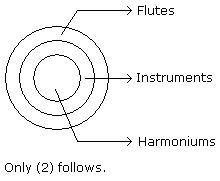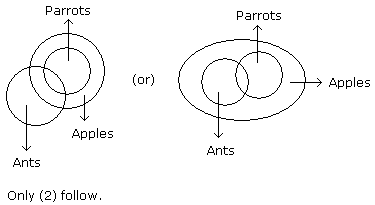# Verbal Reasoning - Syllogism

## Why Verbal Reasoning Syllogism?

In this section you can learn and practice Verbal Reasoning Questions based on "Syllogism" and improve your skills in order to face the interview, competitive examination and various entrance test (CAT, GATE, GRE, MAT, Bank Exam, Railway Exam etc.) with full confidence.

## Where can I get Verbal Reasoning Syllogism questions and answers with explanation?

IndiaBIX provides you lots of fully solved Verbal Reasoning (Syllogism) questions and answers with Explanation. Solved examples with detailed answer description, explanation are given and it would be easy to understand. All students, freshers can download Verbal Reasoning Syllogism quiz questions with answers as PDF files and eBooks.

## Where can I get Verbal Reasoning Syllogism Interview Questions and Answers (objective type, multiple choice)?

Here you can find objective type Verbal Reasoning Syllogism questions and answers for interview and entrance examination. Multiple choice and true or false type questions are also provided.

## How to solve Verbal Reasoning Syllogism problems?

You can easily solve all kind of Verbal Reasoning questions based on Syllogism by practicing the objective type exercises given below, also get shortcut methods to solve Verbal Reasoning Syllogism problems.

### Exercise :: Syllogism - Syllogism 1

In each of the following questions two statements are given and these statements are followed by two conclusions numbered (1) and (2). You have to take the given two statements to be true even if they seem to be at variance from commonly known facts. Read the conclusions and then decide which of the given conclusions logically follows from the two given statements, disregarding commonly known facts.

• (A) If only (1) conclusion follows
• (B) If only (2) conclusion follows
• (C) If either (1) or (2) follows
• (D) If neither (1) nor (2) follows and
• (E) If both (1) and (2) follow.
1.

Statements: Some actors are singers. All the singers are dancers.

Conclusions:

1. Some actors are dancers.
2. No singer is actor.

 A. Only (1) conclusion follows B. Only (2) conclusion follows C. Either (1) or (2) follows D. Neither (1) nor (2) follows E. Both (1) and (2) follow

Explanation:2.

Statements: All the harmoniums are instruments. All the instruments are flutes.

Conclusions:

1. All the flutes are instruments.
2. All the harmoniums are flutes.

 A. Only (1) conclusion follows B. Only (2) conclusion follows C. Either (1) or (2) follows D. Neither (1) nor (2) follows E. Both (1) and (2) follow

Explanation:3.

Statements: Some mangoes are yellow. Some tixo are mangoes.

Conclusions:

1. Some mangoes are green.
2. Tixo is a yellow.

 A. Only (1) conclusion follows B. Only (2) conclusion follows C. Either (1) or (2) follows D. Neither (1) nor (2) follows E. Both (1) and (2) follow

Explanation:4.

Statements: Some ants are parrots. All the parrots are apples.

Conclusions:

1. All the apples are parrots.
2. Some ants are apples.

 A. Only (1) conclusion follows B. Only (2) conclusion follows C. Either (1) or (2) follows D. Neither (1) nor (2) follows E. Both (1) and (2) follow

Explanation:5.

Statements: Some papers are pens. All the pencils are pens.

Conclusions:

1. Some pens are pencils.
2. Some pens are papers.

 A. Only (1) conclusion follows B. Only (2) conclusion follows C. Either (1) or (2) follows D. Neither (1) nor (2) follows E. Both (1) and (2) follow